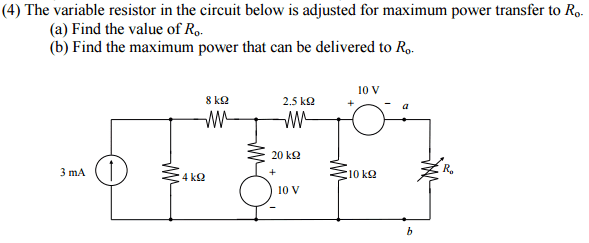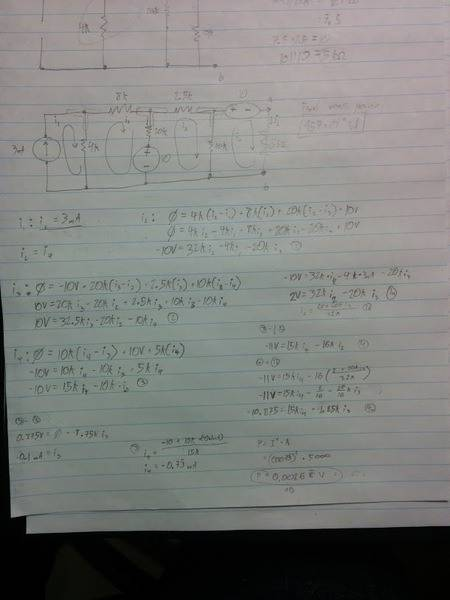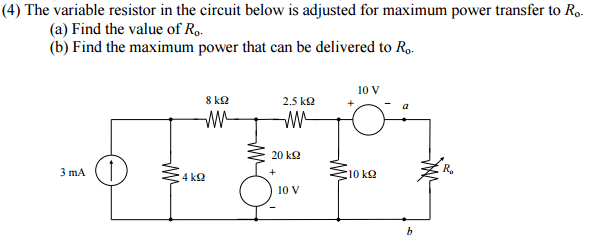# Finding Maximum Power Delivered to Resistor

## Homework StatementPart (a) has already been solved and determined to be 5kΩ, which has been confirmed by the answer key.

The answer key also states that the maximum power able to be delivered to Ro is 957 µW, but doesn't give any workings or explanations on how to reach this answer.

P = I * V
V = I * R
P = I^2 * R

## The Attempt at a Solution

I've attempted to find the power by finding the current across Ro and using that and the value for Ro to find the power. I found the current to be 0.7333 mA using Mesh Current Analysis, but this gives a power of 0.00269 W, which is far from the answer.

My workings are shown in the image below.Sorry if anything is difficult to pick out.

Am I correct in thinking that using Mesh Current to find the current across Ro will work to find the power, or should another circuit analysis method be used?

ehild
Homework Helper

## Homework StatementPart (a) has already been solved and determined to be 5kΩ, which has been confirmed by the answer key.

The answer key also states that the maximum power able to be delivered to Ro is 957 µW, but doesn't give any workings or explanations on how to reach this answer.

P = I * V
V = I * R
P = I^2 * R

## The Attempt at a Solution

I've attempted to find the power by finding the current across Ro and using that and the value for Ro to find the power. I found the current to be 0.7333 mA using Mesh Current Analysis, but this gives a power of 0.00269 W, which is far from the answer.

My workings are shown in the image below.

Sorry if anything is difficult to pick out.

Am I correct in thinking that using Mesh Current to find the current across Ro will work to find the power, or should another circuit analysis method be used?
Yes, you can use the Mesh Current method to find the current across Ro, or you can replace the circuit by its Thevenin equivalent.
Your handwriting is impossible to read, type in your working. Your result is wrong.